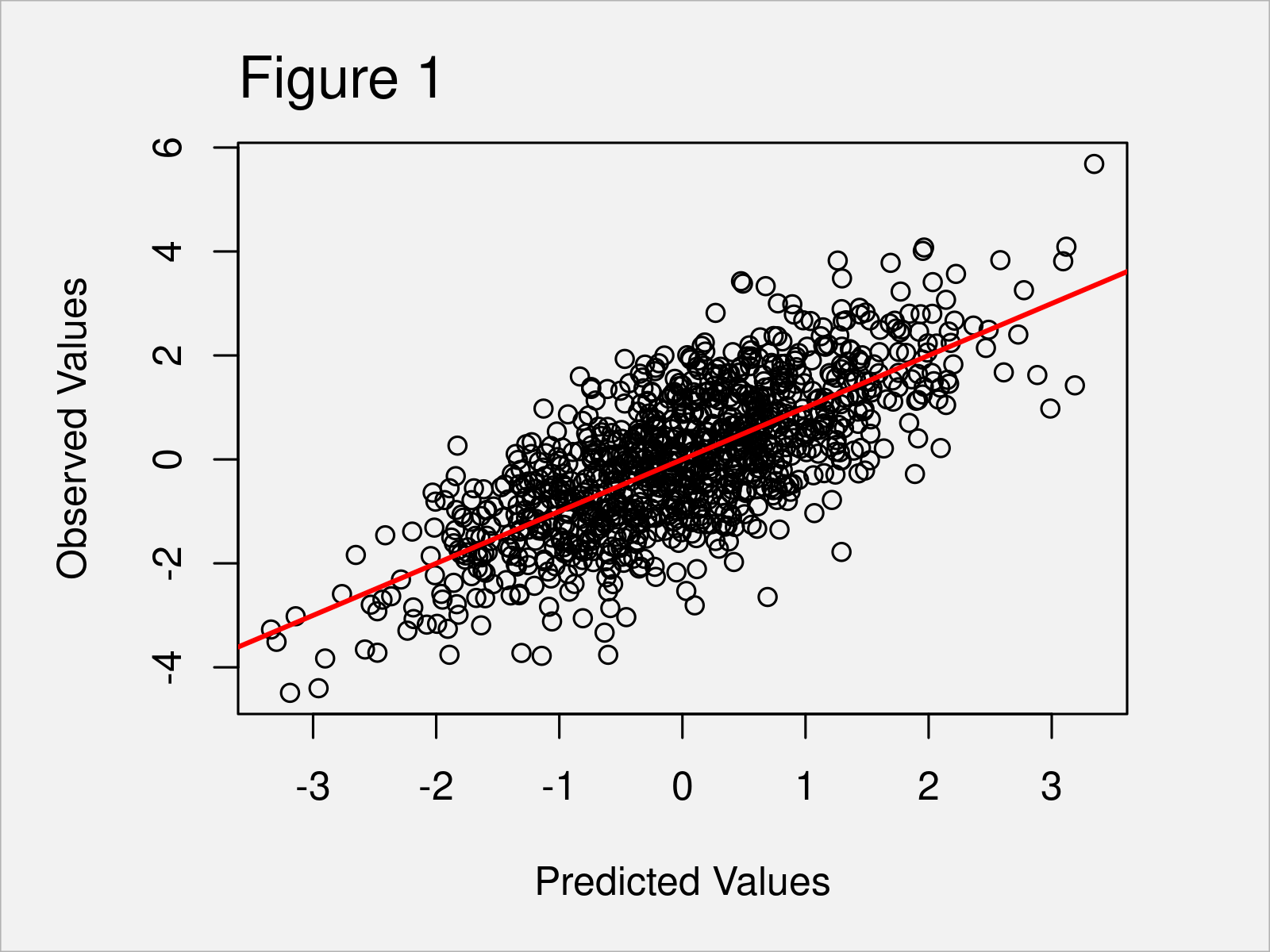# Plot Predicted vs. Actual Values in R (2 Examples)

In this post you’ll learn how to draw a plot of predicted vs. observed values in the R programming language.

The article consists of these contents:

So without further ado, let’s dive into it.

## Creation of Example Data

Consider the following example data.

```set.seed(469827)                                     # Create example data
x <- rnorm(1000)
y <- rnorm(1000) + x
data <- data.frame(x, y)Have a look at the table that got returned after executing the previous R code. It shows the top six rows of our exemplifying data, and that our data has the two numerical columns “x” and “y”.

We can now estimate a linear regression model using the lm function as shown below:

`my_mod <- lm(y ~ x, data)                            # Estimate linear regression model`

The previous R code has created a model object called my_mod. We will use this model to create predicted vs. actual values plots in the following examples.

## Example 1: Draw Predicted vs. Observed Using Base R

This example demonstrates how to plot fitted vs. actual values using the basic installation of the R programming language.

For this, we can use the plot(), predict(), and abline() functions as shown below:

```plot(predict(my_mod),                                # Draw plot using Base R
data\$y,
xlab = "Predicted Values",
ylab = "Observed Values")
abline(a = 0,                                        # Add straight line
b = 1,
col = "red",
lwd = 2)```As shown in Figure 1, we have created a Base R scatterplot that shows predicted vs. actual values. The red line illustrates the slope of our values.

## Example 2: Draw Predicted vs. Observed Using ggplot2 Package

In this example, I’ll demonstrate how to use the ggplot2 package to draw an xy-plot of predicted vs. actual values.

First, we have to install and load the ggplot2 package:

```install.packages("ggplot2")                          # Install & load ggplot2 package
library("ggplot2")```

In the next step, we have to store our predicted and observed values in a new data frame:

```data_mod <- data.frame(Predicted = predict(my_mod),  # Create data for ggplot2
Observed = data\$y)```

Now, we can use this data frame as basis for the creation of our predicted vs. actual values graph:

```ggplot(data_mod,                                     # Draw plot using ggplot2 package
aes(x = Predicted,
y = Observed)) +
geom_point() +
geom_abline(intercept = 0,
slope = 1,
color = "red",
size = 2)```As shown in Figure 2, we have created another fitted vs. actual values graphic. However, this time we have used the ggplot2 package to draw our data.

## Video, Further Resources & Summary

In case you need further info on the topics of this tutorial, you could have a look at the following video on my YouTube channel. I’m demonstrating the content of this article in the video:

Furthermore, you could have a look at the other tutorials on my homepage. Some tutorials are listed below.

Summary: In this tutorial you have learned how to create a scatterplot of predicted vs. observed values in R programming. Tell me about it in the comments section below, in case you have any additional questions.

Subscribe to the Statistics Globe Newsletter

•Shaphan
June 9, 2022 3:12 pm

GREAT VIDEO. THANK YOU FOR SHARING. One Question: What actually are predicted values and how does this information help in understanding and reporting results of a test?

•June 10, 2022 7:13 am